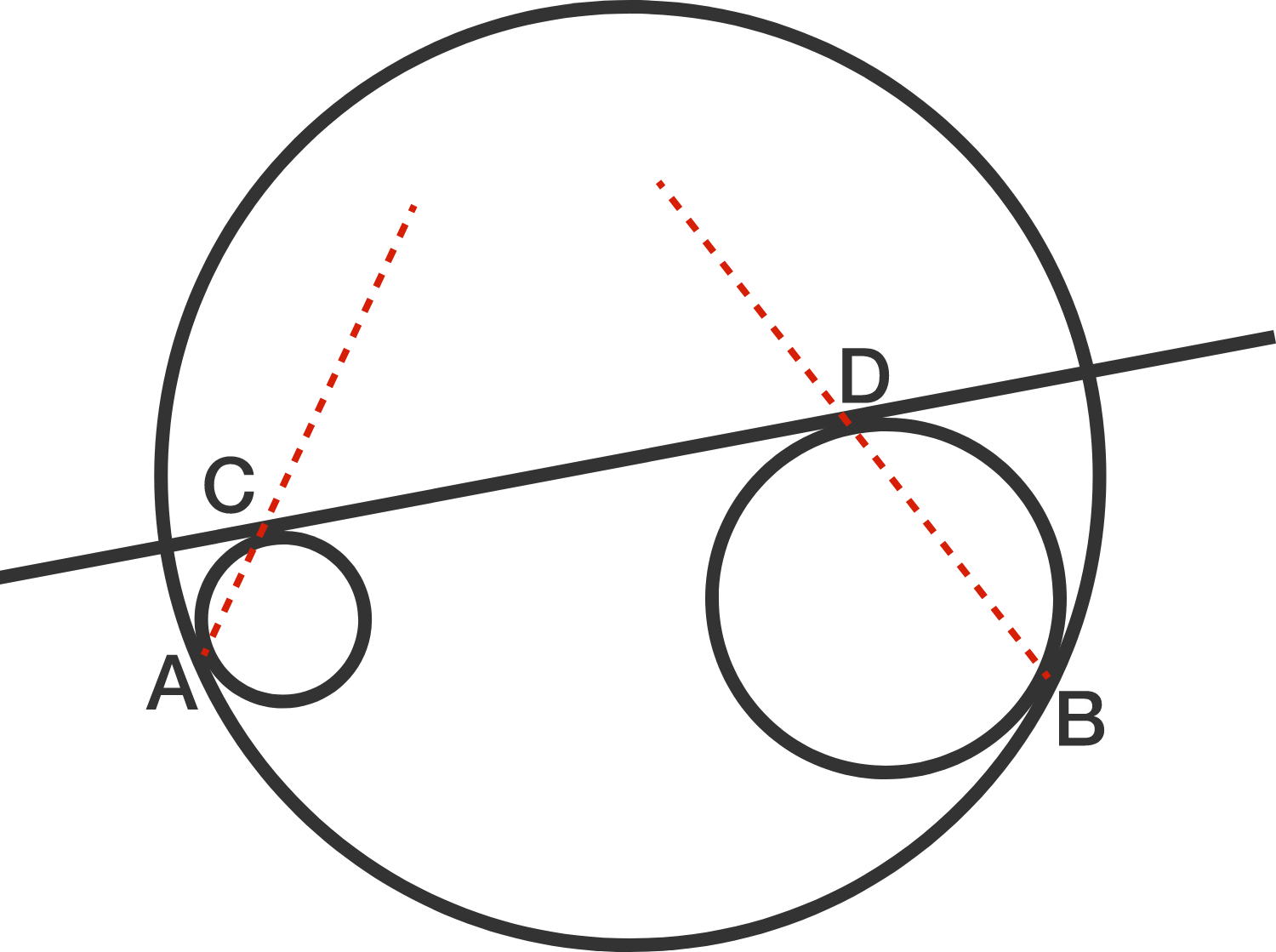# Where Do They Intersect?

Geometry Level 3In a large circle, we draw 2 circles that are each internally tangent at $A$ and $B$. We then draw the common exterior tangent $CD$.

Where do the lines $AC$ and $BD$ intersect?

×

Problem Loading...

Note Loading...

Set Loading...